﻿ Elementary SolutionsISSAC 94 - Symbolic-Numeric Nonlinear Equation Solving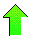Contents News View

 Elementary Solutions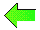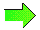Bundy and Welham describe concepts of attraction, collection, and isolation which sometimes lead to solutions of nonlinear equations.  Taking a somewhat related algorithmic approach, suppose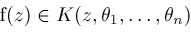is an elementary expression where each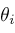is exponential, logarithmic, or algebraic.  Factoring, we suppose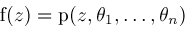is irreducible in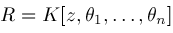.  Apply rules

(1)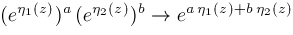(2)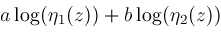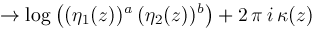(3)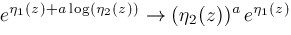(4)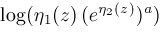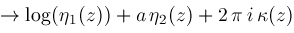(5)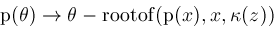(6)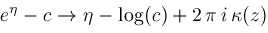(7)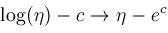(8)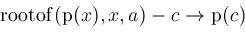where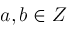and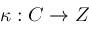is an integer-valued piecewise constant function of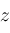For rule (1), letand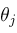be exponentials.  Try to find integers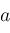,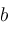,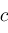such that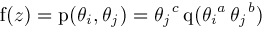(consider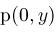and degrees) and simplify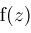to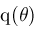where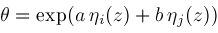For rule (2), letandbe logarithms.  Try to find a rational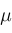such that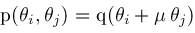(consider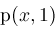,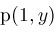, and lcoeff's) and simplifyto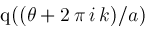where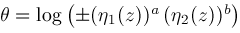,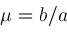, and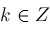is chosen according to a given root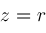(given by our numerical algorithm).

Rules (1)-(2) are applied first.  Rules (3)-(8) are applied with new factorization.  This scheme will solve some equations.